# Intermediate Geometry : Sectors

## Example Questions

← Previous 1 3 4 5 6 7 8 9 13 14

### Example Question #1 : Intermediate Geometry

How many degrees are in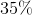of a circle?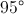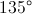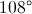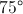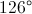Explanation:

There are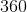degrees in a circleso the equation to solve becomes a simple percentage problem: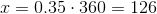### Example Question #1 : How To Find The Angle For A Percentage Of A Circle

A sector contains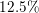of a circle.  What is the measure of the central angle of the sector?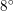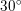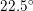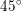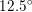Explanation:

An entire circle is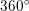. A sector that isof the circle therefore has a central angle that isof.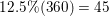Therefore, our central angle is### Example Question #1 : How To Find The Angle For A Percentage Of A Circle

If you have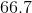percent of a circle, what is the angle, in degrees, that creates that region?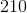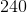Explanation:

A full circle has 360 degrees, which means that 100% of the circle is 360 degrees.

Now you need to convert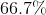into a decimal.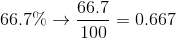If you multiply 360 by 0.667, you get the degree measure that corresponds to the percentage, which is 240.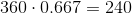### Example Question #1 : Sectors

If you haveof a circle, what is the angle, in degrees, that creates that region?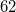Explanation:

A full circle has 360 degrees, which means that 100% of the circle is 360 degrees.

First convertinto a decimal.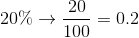If you multiply 360 by 0.20, you get the degree measure that corresponds to the percentage, which is 72.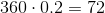### Example Question #5 : Intermediate Geometry

If you have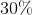of a circle, what is the angle, in degrees, that creates that region?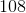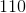Explanation:

A full circle has 360 degrees, which means that 100% of the circle is 360 degrees.

In order to start this problem we need to convert the percent into a decimal.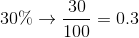If you multiply 360 by 0.30, you get the degree measure that corresponds to the percentage, which is 108.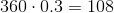### Example Question #6 : Intermediate Geometry

If you haveof a circle, what is the angle, in degrees, that creates that region?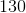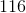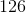Explanation:

A full circle has 360 degrees, which means that 100% of the circle is 360 degrees.

First convert the percent to decimal.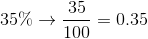Now if you multiply 360 by 0.35, you get the degree measure that corresponds to the percentage, which is 126.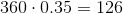### Example Question #1 : How To Find The Angle For A Percentage Of A Circle

If you have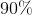of a circle, what is the angle, in degrees, that creates that region?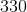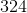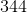Explanation:

A full circle has 360 degrees, which means that 100% of the circle is 360 degrees.

First convert the percentage into a decimal.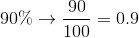If you multiply 360 by 0.90, you get the degree measure that corresponds to the percentage, which is 324.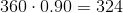### Example Question #8 : Intermediate Geometry

If you have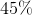of a circle, what is the angle, in degrees, that creates that region?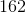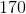Explanation:

A full circle has 360 degrees, which means that 100% of the circle is 360 degrees.

First we need to convert the percentage into a decimal.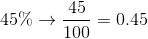If you multiply 360 by 0.45, you get the degree measure that corresponds to the percentage, which is 162.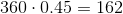### Example Question #1 : How To Find The Angle For A Percentage Of A Circle

If you have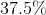of a circle, what is the angle, in degrees, that creates that region?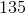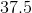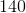Explanation:

A full circle has 360 degrees, which means that 100% of the circle is 360 degrees.

In order to solve this problem we first need to convert the percentage into a decimal.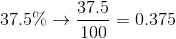If you multiply 360 by 0.375, you get the degree measure that corresponds to the percentage, which is 135.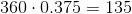### Example Question #10 : Intermediate Geometry

If you haveof a circle, what is the angle, in degrees, that creates that region?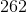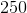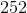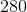Explanation:

A full circle has 360 degrees, which means that 100% of the circle is 360 degrees.

First we need to convert the percentage into a decimal.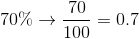If you multiply 360 by 0.70, you get the degree measure that corresponds to the percentage, which is 252.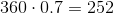← Previous 1 3 4 5 6 7 8 9 13 14

### All Intermediate Geometry Resources